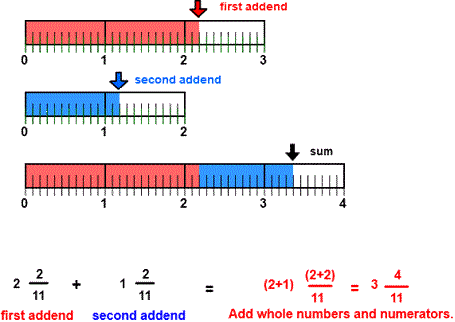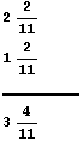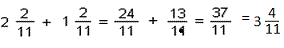CORRECT:
ATTEMPTS:
SCORE:
PERCENT
WHOLE
NUMERATOR
DENOMINATOR

# ADDITION OF LIKE FRACTIONS INSTRUCTIONS

Follow the directions in the dialog box after pressing the <START> button. The <EXPLAIN> button may be pressed after you enter the second addend to see how to do the example.

When the program starts, you will be asked to identify the first addend and then the second addend. The program will not continue unless each addend is correctly identified. You will then be asked to add each addend for the sum.You can see from the picture that the first addend is 2 complete units and 211 partial units in length and the second addend is 1 complete unit and 211 partial units in length. The sum will combine the complete units for 3 complete units and the partial units for 411 partial units for a sum of 3 411 units.

In many examples, the sum may be found by visually combining the two addends. In the picture you can see that you have two complete units in the first addend and one complete units in the second addend for three complete units.

To calculate the sum first add the whole numbers 2 and 1 to obtain 3 for a whole number. Since the denominators of the fractions 211 and 211 are the same you can add the numerators of the to obtain the 411 in the sum. Fractions with the same denominators are like fractions.

You may prefer to work vertically:or you may prefer to write each addend in fraction form first:You may enter the sum in fraction form or mixed number form. In the above example 3711 or 3 411 are both acceptable.

For more instruction on adding fractions go to How To Add Fractions.

After you enter the sum you may press the <REPORT> button. The report will ask for your name but you may submit a code for your name. This report will give the same results as on the dialog box. The report may be printed or e-mailed.